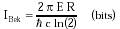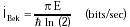Nanomedicine, Volume I: Basic Capabilities

Robert A. Freitas Jr., Nanomedicine, Volume I: Basic Capabilities, Landes Bioscience, Georgetown, TX, 1999

10.2.4.3 Bekenstein-Bounded Computation

A fundamental upper limit on the number of possible quantum states in a bounded region (e.g., the maximum number of bits that can be coded in a bounded region) -- is given by the Bekenstein Bound1990 as:{Eqn. 10.10}

within a spherical region of radius R containing energy E = mc2 where m is the enclosed mass, c = 3 x 108 m/sec (speed of light), and h = h / 2 p where h = 6.63 x 10-34 joule-sec (Planck's constant). Maximum processing speed 'I is then bounded by the minimum time for a state transition, which cannot be less than the time required for light to cross the region of radius R, or:{Eqn. 10.11}

Thus in theory, a single carbon atom of mass m = 2 x 10-26 kg and radius R ~ 0.15 nm could be impressed with up to IBek ~ 108 bits and could process information up to 'IBek ~ 1026 bits/sec in an optimally-designed quantum computer. For a 1 micron3 computer of mass ~10-15 kg, IBek ~ 1022 bits maximum storage capacity and 'IBek ~ 1037 bits/sec maximum processing capacity. These values are not the least upper limits; Schiffer and Bekenstein1991 estimate that the Bekenstein Bound probably overestimates I and 'I by at least a factor of 100, but Likharev1992 and Margolus and Levitin2319 have derived similar limits. In 1998, proposed reversible quantum computer systems would perform significantly below the Bekenstein Bound.1993,1994

Last updated on 24 February 2003